Bayesian Linear Regression

Univariate Gibbs Sampler

• R script: myGibbs.txt
• Literature 1: Mrode, R.A. 2005. Linear Models for the Prediction of Animal Breeding Values. CAB International, Oxon, UK.
• Literature 2: Misztal, I. 2008. Computational Techniques in Animal Breeding. Course Notes. University of Georgia.
1. Given the components of MME, use Gibbs sampling. Consider the following univariate mixed linear model.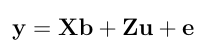In the Mixed Model Equation form: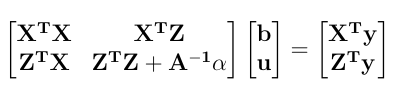where 'A' is an additive relationship matrix and alpha is a ratio of the variance components. It can also be written as: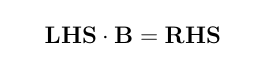Univariate Gibbs sampling can be performed by taking samples from following distribution.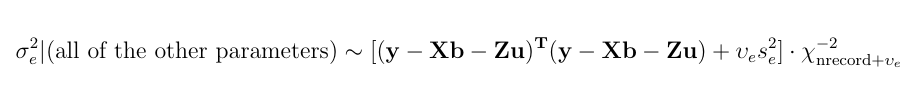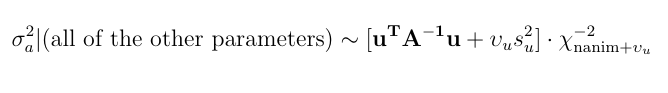2. Here is a numerical application from Mrode (2005), example 12.1, which deals with the pre-weaning gain (WWG) of beef calves.
> # response
> y <- c(4.5,2.9,3.9,3.5,5.0)
>
> # pedigree
> s <- c(0,0,0,1,3,1,4,3)
> d <- c(0,0,0,0,2,2,5,6)
> Ainv <- quass(s,d)
>
> # X matrix
> X <- matrix(c(1,0,0,1,1,0,1,1,0,0), nrow=5, ncol=2)
> X
[,1] [,2]
[1,]    1    0
[2,]    0    1
[3,]    0    1
[4,]    1    0
[5,]    1    0
> # Z matrix
> z1 <- matrix(0, ncol=3, nrow=5)
> z2 <- matrix(c(1,0,0,0,0,0,1,0,0,0,0,0,1,0,0,0,0,0,1,0,0,0,0,0,1), ncol=5)
> Z <- cbind(z1,z2)
> Z
[,1] [,2] [,3] [,4] [,5] [,6] [,7] [,8]
[1,]    0    0    0    1    0    0    0    0
[2,]    0    0    0    0    1    0    0    0
[3,]    0    0    0    0    0    1    0    0
[4,]    0    0    0    0    0    0    1    0
[5,]    0    0    0    0    0    0    0    1

Set the initials of B (b and u) to zero, the environmental variance to 40, the additive genetic variance to 20 and assume "naive" ignorance improper priors for the variance components such that ve = va = 0 and s2e = s2e = 0
> inits <- c(0,0,0,0,0,0,0,0,0,0)
> varE <- 40
> varA <- 20
> ve <- 0
> va <- 0
> s2e <- 0
> s2a <- 0

Further, allow 2000 "burnin" samples. Then collect 5000 samples after the burnin period. Define the disp option as TRUE.
> burnin <- 2000
> N <- 5000
> disp <- TRUE

3. To get the complete result, let's run myGibbs function.
> myGibbs(Ainv, y, X, Z, N, burnin, inits, varE, varA, ve, va, s2e, s2a, disp)

iteration  1
varA  27.90349
varE  9.830295

iteration  2
varA  27.62676
varE  6.469905

iteration  3
varA  3.537537
varE  1.264450

.
.
.
snip
.
.
.

iteration  6998
varA  0.08454485
varE  0.2756133

iteration  6999
varA  0.3397200
varE  0.5079951

iteration  7000
varA  0.6410488
varE  0.336344

\$`Solutions`
  4.42481745  3.46324977  0.04799799 -0.07124684 -0.06597394 -0.05571167
 -0.23979331  0.11705991 -0.30874805  0.12379153

\$`Variance Components`
 1.269034 2.032178

Solutions and Solutionss are sex effects. Prediction through Prediction are predicted breeding values. The first variance component is an estimate of the additive genetic variance and the second one is an estimate of the envirommental variance.